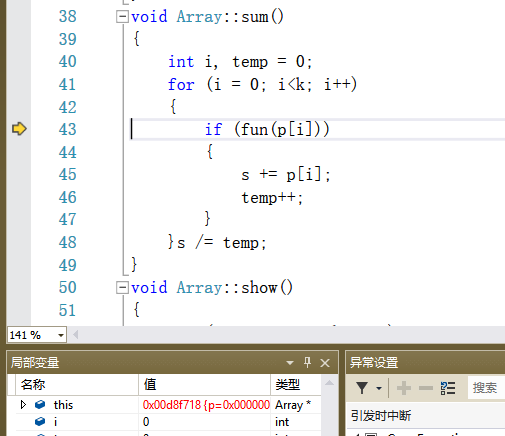C++题目，求教，输出不了结果。

（1）私有成员数据： int *p,k; // p 表示维组，k 为数组大小 float *s; //维组中素数元素的平均值

（2）公有成员函数： Array(int*ptr,int n)：根据参数初始化 p 和 k，然后初始化 s 并为 s 赋初值；

int fun(int n)：判断 n 是否为到数，若是返回 1，否则返回 0；

void sum ( )：求素数元素的平均值；

void show( )：输出所有成员数据，数组每行 5 个元素，其他成员每行一个； 析构函数。

（3）设计一个完整的程序对该类进行测试。 以数组{5,2,7,4,8,23,65,1,40}说明 Array 类型对象 arr，并对其测试。

``````#include<iostream>
using namespace std;
class Array
{
private:
int *p,k;
float s;
public:
Array(int *ptr,int n);
int fun(int n);
void sum();
void show();
~Array();
};
Array::Array(int *ptr,int n)
{
k=n;
int *p=new int[k];
for(int i=0;i<k;i++)
p[i]=ptr[i];
s=0;
}
int Array::fun(int n)
{
if(n<2) return 0;
for(int i=2;i<n;i++)
if(n%i==0) return 0;
return 1;
}
void Array::sum()
{
int i,temp=0;
for(i=0;i<k;i++)
{
if(fun(p[i]))
{
s+=p[i];
temp++;
}
}s/=temp;
}
void Array::show()
{
for(int i=0;i<k;i++)
{
cout<<p[i]<<" ";
if(i%4==0)cout<<'\n';
}
cout<<"\n数组大小为"<<k<<"\n其中素数的平均数为:"<<s<<endl;
}
Array::~Array(){delete []p;}
int main()
{
int a[]={5,2,7,4,8,23,65,1,40};
Array arr(a,9);
arr.sum();
arr.show();
return 0;
}
``````

1个回答